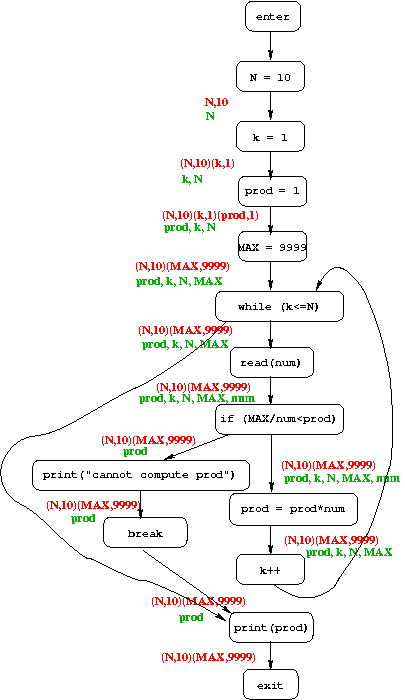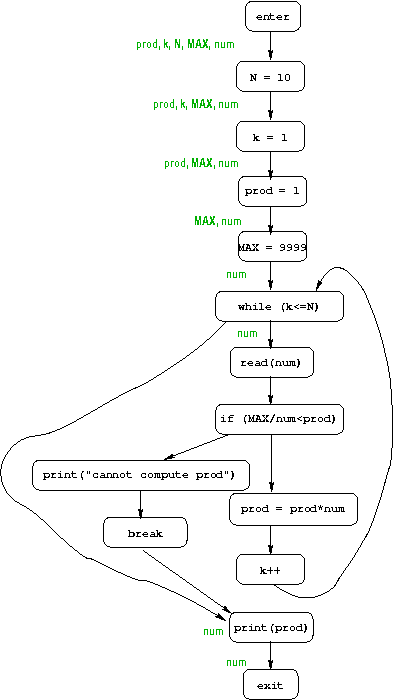### Test Yourself #1

The constant-propagation information is in red, and the live-variables information is in green.### Test Yourself #2

The definition of the may be uninitialized problem is as follows:

• An individual dataflow fact is a set of variables (those that might be uninitialized), so the domain D of dataflow facts is the set of all sets of variables.
• The combining (meet) operator is set union.
• The initial dataflow fact (what is true at the entry node) is the set of all variables in the program.
• This is a GEN/KILL problem, so for each CFG node n, the dataflow function is of the form fn(S) = S - KILLn union GENn. The KILL set for node n is the set of variales defined at n, and the GEN set is always empty.### Test Yourself #3

Here are the dataflow equations for live analysis. Note that the meet operator (⌈⌉) is set union.
enter.after = 1.before
1.before = 1.after - {x}
1.after = 2.before
2.before = 2.after - {y} union {x}
2.after = 3.before
3.before = 3.after
3.after = exit.before ⌈⌉ 4.before
4.before = 4.after union {y}
4.after = 3.before exit.before = empty-set

 Variable Least Solution Greatest Solution 1.before { } { } 1.after { x } { x } 2.before { x } { x } 2.after { y } { x, y} 3.before { y } { x, y} 3.after { y } { x, y } 4.before { y } { x, y} 4.after { y } { x, y }

The solution we want is the least solution.